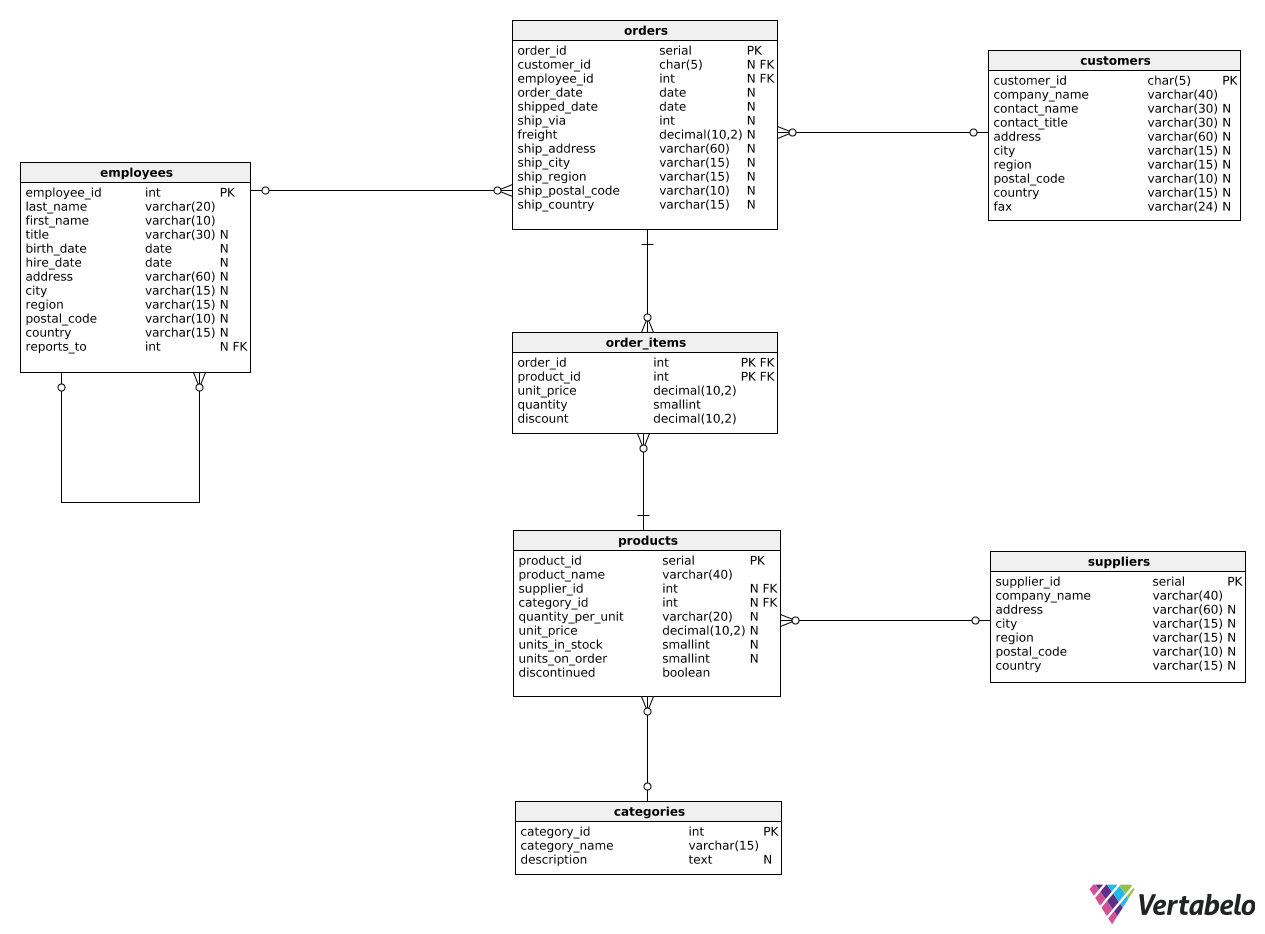Deals Of The Week - hours only!Up to 80% off on all courses and bundles.-Close
Introduction
Multiple metrics for a single object
Metrics for two groups
Ratios and percentages
6. Calculating ratios – step 1
Global vs. specific metrics
Summary

## Instruction

Excellent! How can we compute percentages and ratios in our reports? Let's find out.

Suppose we want to know what percentage of all orders have already been shipped. We'll write this query in a few steps. Here's step 1:

SELECT
COUNT(CASE
WHEN shipped_date IS NOT NULL
THEN order_id
END) AS count_shipped,
COUNT(order_id) AS count_all
FROM orders;


In this step, we simply calculate two separate columns: the numerator and the denominator of our ratio. In this case, the numerator is the number of orders shipped and the denominator is the total number of orders.

Note how we used COUNT() with CASE WHEN to compute one metric (number of orders shipped) and COUNT() to compute the other metric (total number of orders).

## Exercise

We want to find the ratio of the revenue from all discounted items to the total revenue from all items. We'll do this in steps too.

First, show two columns:

1. discounted_revenue – the revenue (after discount) from all discounted line items in all orders.
2. total_revenue – the total revenue (after discount) from line items in all orders.

### Stuck? Here's a hint!

You only need the order_items table. Use the discount column to determine whether a given line item was discounted.

You can calculate total revenue as follows:

SUM(unit_price * quantity * (1 - discount))